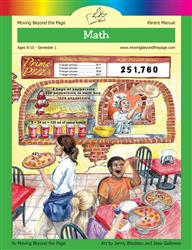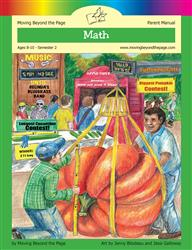Age 5-7 Reading Is Now Available!

# Common Core Alignment

CCSS.Math.Content.4.NBT.6 - Find whole-number quotients and remainders with up to four-digit dividends and one-digit divisors, using strategies based on place value, the properties of operations, and/or the relationship between multiplication and division. Illustrate and explain the calculation by using equations, rectangular arrays, and/or area models.

## 5: MathUnit 4: Multi-Digit Multiplication
Lesson 4: Multi-Digit Multiplication Using Arrays
Lesson 5: The Area Model
Lesson 6: The Standard Multiplication Algorithm
Lesson 7: Multiplication Practice
Lesson 8: Unit Test
Final Project: Multiplication ContractUnit 6: Multi-Digit Division
Lesson 2: Dividing Within 100
Lesson 3: Getting Started With Long Division
Lesson 4: Long(er) Division
Lesson 5: Division Problem Solving
Lesson 6: Unit Test
Final Project: Long Division Poster